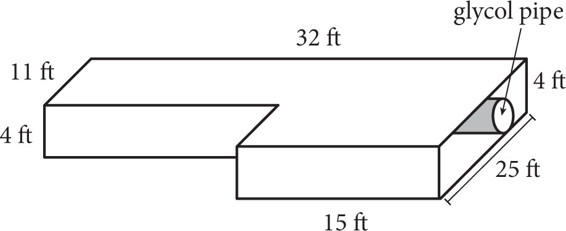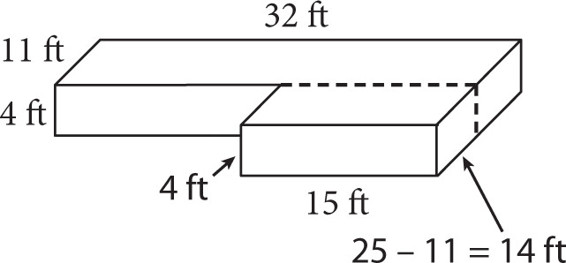# SAT Math Multiple Choice Question 371: Answer and Explanation

### Test Information

Question: 371

11. A zoo is building a penguin exhibit. It will consist of an underwater area and a land area. The land area is made of thick sheets of ice. An outline of the total space covered by the ice is shown below. A pipe 2 feet in diameter runs the full length of the exhibit under the ice. A substance known as ice-cold glycol continuously runs through the pipe to keep the ice frozen.About how many cubic feet of water are needed to create the ice portion of the exhibit?

• A. 1,850
• B. 2,150
• C. 2,450
• D. 3,100

Explanation:

B

Difficulty: Medium

Category: Additional Topics in Math / Geometry

Strategic Advice: The amount of water needed to create the ice portion of the exhibit is another way of saying the volume of the ice. So, you need to find the volume of the entire space and then subtract the volume of the cylinder that runs through the ice. The volume of a rectangular prism is given by V = l × w × h, and the volume of a cylinder equals the area of its base times its height, or πr2h.

Getting to the Answer: To determine the volume of the ice, start by decomposing the figure into two rectangular prisms and adding their volumes. You can decompose the figure left to right or front to back. Front to back, it looks like the following figure:The prism in the back has a volume of 32 × 11 × 4 = 1,408 cubic ft. The prism in the front has a length of 15 ft and a height of 4 ft, but the width is missing. Find the missing width by subtracting 11 from 25, which is 14 ft. So, the volume of the prism in the front is 15 × 14 × 4 = 840 cubic ft. The total volume of the prisms is 1,408 + 840 = 2,248 cubic ft. Be careful—that's not the answer. You still need to find the amount of space taken up by the glycol pipe and subtract it. The diameter of the pipe is 2 ft, so its radius is 1 foot, and the height (or the length in this question) is 32 ft, so the volume is π(1)2 (32) ≈ 100.53 cubic ft. This means the amount of ice needed is 2,248 – 100.53 = 2,147.47, or about 2,150 cubic ft.